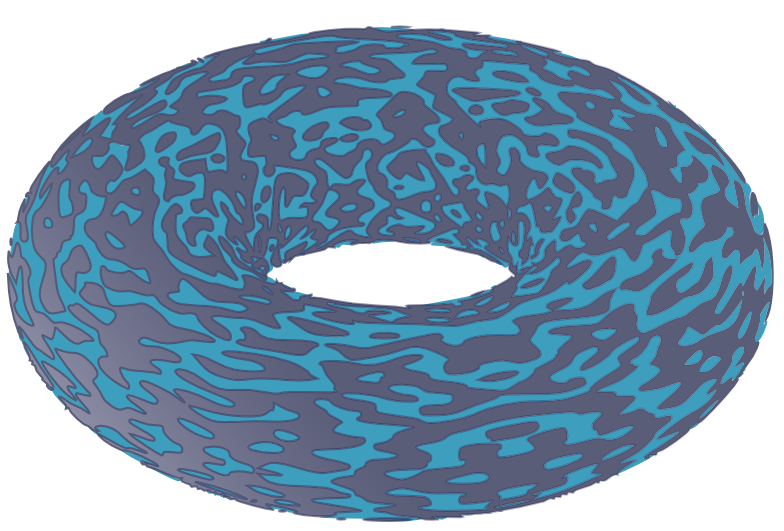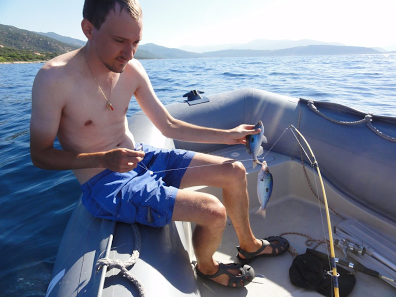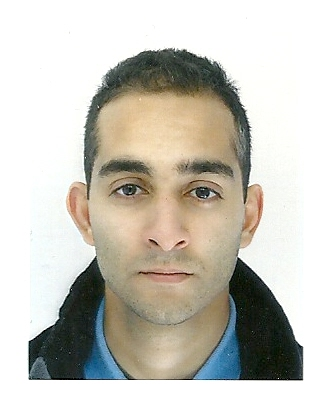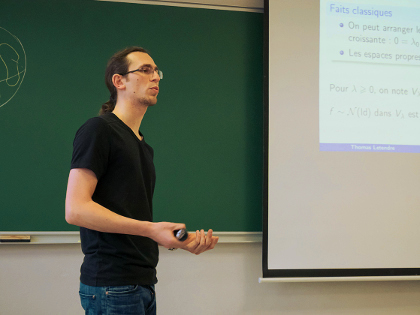UNIRANDOM is four year long scientific project starting in January 2018, financed by the French ANR agency (Agence Nationale de la Recherche) in the framework of the national program ANR JCJC. The project is coordinated by Guillaume Poly in the University of Rennes 1 and gathers five young researchers with a common solid background in probability theory but also complementary domains of expertise. As the logo above suggests, the acronym UNIRANDOM stands for Universality for random nodal domains.

DESCRIPTION OF THE PROJECT

Nodal sets, i.e. vanishing loci of functions, are central objects in mathematics. Understanding the main features of a purely deterministic nodal set is generally out of reach, as illustrated by several celebrated open problems, such as Hilbert’s sixteenth problem or Yau’s conjecture. In order to capture the typical behavior of an object, one is thus tempted to randomize, which reduces here to consider nodal sets associated to random functions. Computing expected values, variances or else fluctuations around the mean of the considered nodal functionals, and in particular understanding their asymptotic behavior as the amount of noise goes to infinity, is then a true wealth of information about the possible deterministic behaviors. Besides, randomization of nodal sets is also strongly motivated by deep physical insights, such as the celebrated Berry’s conjecture.

In this framework, the project UNIRANDOM is mainly focused on universality results, that is, asymptotic properties of random level sets, holding regardless of the specific nature of the randomness involved. Establishing such universal properties for random zero sets allows one to manage what would be otherwise inextricable objects in a purely deterministic setting, which explains the tremendous importance of this area of research.

The project concentrates on four main multidimensional models of random nodal sets which find their roots in various and rich domains of mathematics and physics, namely: A. Nodal domains associated to random eigenfunctions on generic Riemannian compact manifolds, B. Arithmetic random waves, C. Random algebraic manifolds, D. Periodic random fields. This project is thus intrinsically multidisciplinary and brings together five young researchers with a common solid background in probability theory but also complementary domains of expertise. Furthermore, our project is particularly ambitious and innovative since the question of universality, although well understood for several models in dimension one, has hardly been investigated in multidimensional frameworks.

MEMBERS OF THE PROJECT

Guillaume PolyG. Poly defended his Ph.D. in 2011 at Ecole nationale des ponts et chaussées, under the supervision of N. Bouleau, in the domain of Dirichlet forms and potential analysis. During 2 years of post-doc, he broadened his research scope, at Université du Luxembourg, in the team of G. Peccati by working on Malliavin calculus and Stein’s method. Since Fall 2014, he is maître de conférences (assistant professor) at Université de Rennes 1. This fresh permanent position is the perfect opportunity for him to begin long and very ambitious projects. It is also the right time to endorse more responsibilities, organize events and interact with researchers working on thriving domains.

Jürgen AngstSince 2010, Jürgen Angst is maître de conférences at the Université de Rennes 1. Appart from his recent interest in random nodal domains, his main research area lies between probability theory, differential geometry and mathematical physics. It consists in studying the links between the geometry of pseudo-Riemannian manifolds, especially Lorentzian manifolds, and the behavior of random curves drawn on these manifolds. He therefore has a strong background in probability theory as well as in differential geometry, harmonic analysis and mathematical physics. Thanks to his geographical proximity with G. Poly, their collaboration will be particularly active.

Rafik ImekrazSince 2012, R. Imekraz is maître de conférences at the University of Bordeaux. His first research interest deals with partial differential equations and more precisely with the long time existence for solutions to Klein-Gordon or Schrödinger equations. Since 2015, he are also interested in the connection between probability and mathematical physics, e.g. the use of probabilistic methods to exhibit Hilbert basis that display unexpected behaviors. his main point of interest in this project is the extension of classical theorems known for explicit Laplacian eigenfunctions on the torus to other linear models whose eigenfunctions are not explicit (Riemannian compact manifolds or harmonic oscillator).

Thomas LetendreThomas Letendre is post-doc at Sorbonne University, Paris. In November 2016, he defended his thesis whose subject is the geometry and topology of random sub-manifolds, both in a Riemannian setting and in a real algebraic one. He is also familiar with a related model of complex random algebraic sub-manifolds in a polarized Kähler manifold as well as the theory of Hörmander's peak sections. One of his interests lies in the relation between the geometry of random sub-manifolds and that of the ambient space. He has a strong background in Riemannian and smooth algebraic geometry, both real and complex, as well as probability theory.

Maurizia RossiMaurizia Rossi is Assistant Professor at University of Pisa. Before that, she was post-doc at Université Paris-Descartes and Research Associate in the probability group of G. Peccati at the University of Luxembourg. She received her Ph.D. in mathematics from the University of Rome Tor Vergata in November 2015, defending the thesis ''The geometry of spherical random fields'' under the supervision of P. Baldi and D. Marinucci. She is mainly interested in probability theory and its interactions with other disciplines, such as geometry, algebra and analysis. Her main research topics concern the geometry of random eigenfunctions on Riemannian manifolds, properties of invariant random fields on algebraic structures, large deviations for diffusion processes and applications, and probabilistic approximations.

PUBLICATIONS

R. Imekraz, Multidimensional Paley-Zygmund theorems and sharp Lp estimates for some elliptic operators,
To be published in Annales de l'Institut Fourier
https://hal.archives-ouvertes.fr/hal-01572331

J. Angst and G. Poly, On the absolute continuity of random nodal volumes,
Submitted
https://arxiv.org/abs/1811.04795

V. Bally, L. Caramellino,and G. Poly, Non universality for the variance of the number of real roots of random trigonometric polynomials,
Probability Theory and Related Fields

J. Angst, F. Dalmao and G. Poly, On the real zeros of random trigonometric polynomials with dependent coefficients,
Proc. Amer. Math. Soc. 147 (2019), 205-214
http://www.ams.org/journals/proc/2019-147-01/S0002-9939-2018-14216-1/

J. Angst, V.H. Pham and G. Poly, Universality of the nodal length of bivariate trigonometric polynomials,
Trans. Amer. Math. Soc (2018),
https://doi.org/10.1090/tran/7255

T. Letendre, Variance of the volume of random real algebraic submanifolds,
Trans. Amer. Math. Soc. (2018),
https://doi.org/10.1090/tran/7478

T. Letendre et M. Puchol, Variance of the volume of random real algebraic submanifolds II,
Indiana Univ. Math. J., to be published
arXiv:1707.09771

R. Imekraz, Concentration et randomisation universelle de sous-espaces propres
Analysis & PDE (2018), 11(2), pages 263-350,
https://projecteuclid.org/euclid.apde/1513774507

F. Dalmao, I. Nourdin, G. Peccati, M. Rossi: Phase singularities in complex arithmetic random waves.
Electronic Journal of Probability (in press).
http://arxiv.org/abs/1608.05631

I. Nourdin, G. Peccati, M. Rossi: Nodal statistics of planar random waves.
Communications in Mathematical Physics (in press).
https://doi.org/10.1007/s00220-019-03432-5

D. Marinucci, M. Rossi, I. Wigman: The asymptotic equivalence of the sample trispectrum and the nodal length for random spherical harmonics.
Annales de l’Institut Henri Poincaré, Probabilités et Statistiques (in press).
https://arxiv.org/pdf/1705.05747.pdf

M. Rossi: The defect of random hyperspherical harmonics.
Journal of Theoretical Probability (in press).

M. Rossi, I. Wigman: Asymptotic distribution of nodal intersections for arithmetic random waves.
Nonlinearity, 31, 4472 (2018).
http://iopscience.iop.org/article/10.1088/1361-6544/aaced4

G. Peccati, M. Rossi: Quantitative limit theorems for local functionals of arithmetic random waves.
Computation and Combinatorics in Dynamics, Stochastics and Control, The Abel Symposium, Rosendal, Norway, August 2016, 13, 659-689 (2018).
https://www.springer.com/gp/book/9783030015923#

S. Campese, D. Marinucci, M. Rossi: Approximate normality of high-energy hyperspherical eigenfunctions.
Journal of Mathematical Analysis and Applications, 461, 1, 500–522 (2018).
https://www.sciencedirect.com/science/article/pii/S0022247X17310521

J. Angst and G. Poly, On the Pulperia theorem for dependant variables
arXiv:1802.007

EVENTS - CONFERENCE 2019

Within the four years of the project UNIRANDOM, we plan to organize several workshops and two international conferences. The first international conference will take place in Rennes, on the week 9-13 September 2019. It will gather specialists from various mathematical disciplines such as algebraic geometry, spectral analysis, probability theory or else mathematical physics that share a common interest in the study of random nodal domains and their geometric, analytic or probabilistic properties.

The participants will expose their last results on the subject during 40 minutes talks. We will favour discussions and networking in a friendly atmosphere to encourage future collaborations.